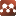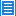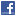## Relativity and Cosmology## E=mc2 Dimensional Descriptor Usage Error

Authors: Frank H. Makinson

E=mc2 is Einstein's mass-energy equation. It is an algebraic equation that uses letters to represent single or composite numbers. Each number type on the right side of the equal sign, mass, distance and a unit time duration are defined as System International basic units. As described, they correctly represent a dimension type. Over a centuries ago, the unit time duration dimension, the second, was improperly used to represent the time duration for the longest wavelength an electromagnetic wave can have and it would have a frequency of “one”. As a result, all current physical law equations that contain a value for electromagnetic wave frequency or the speed of light are using a non-linear scale.